Question

# Draw the shear and bending-moment diagrams for the beam and loading shown.

Draw the shear and bending-moment diagrams for the beam and loading shown. Determine the maximum absolute value (a) of the shear, (b) of the bending moment.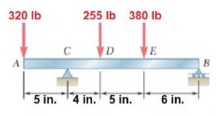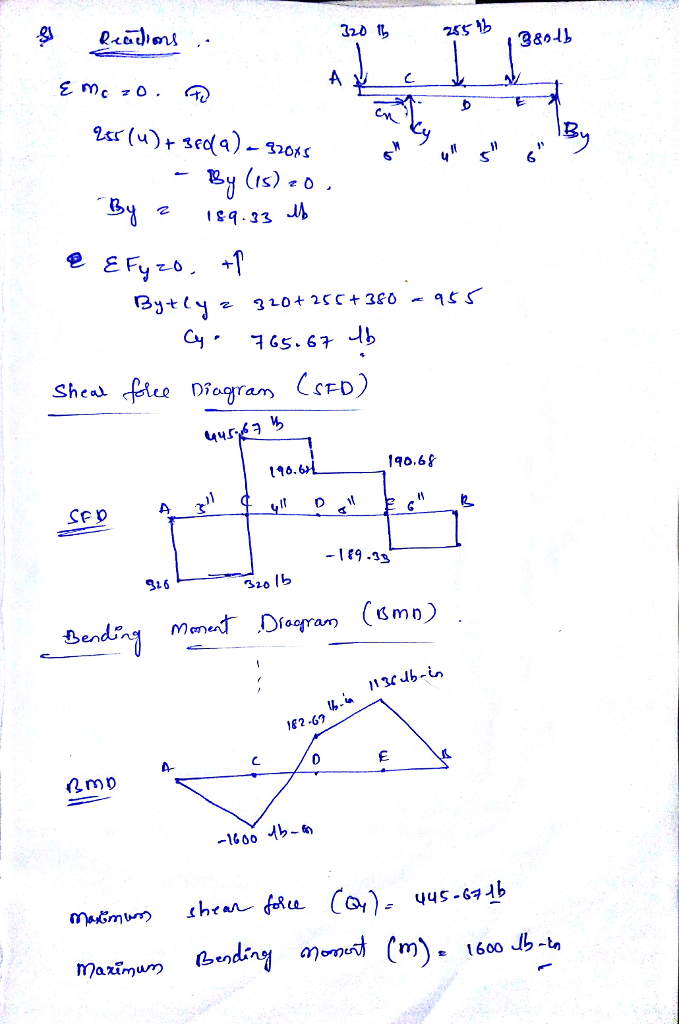#### Earn Coins

Coins can be redeemed for fabulous gifts.

Similar Homework Help Questions
• ### Draw the shear and bending-moment diagrams for the beam and loading shown.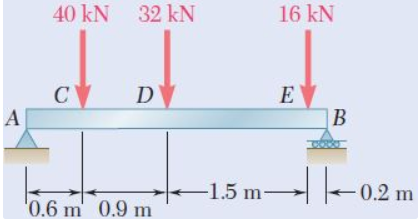Required information Consider the given beam and loading. Draw the shear and bending-moment diagrams for the beam and loading shown.Determine the maximum absolute values of the shear and bending moment. (Round the final answer to one decimal place.) The maximum absolute shear force is _______ KN. The maximum absolute bending moment is _______ kN.m.

• ### For the beam and loading shown, (a) draw the shear and bending-moment diagrams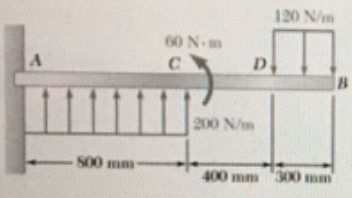For the beam and loading shown, (a) draw the shear and bending-moment diagrams, (b) determine the maximum absolute values of the shear and bending moment.

• ### For the beam and loading shown, (a) draw the shear and bending-moment diagrams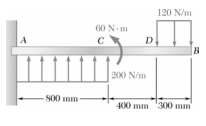For the beam and loading shown, (a) draw the shear and bending-moment diagrams, (b) determine the maximum absolute values of the shear and bending moment.

• ### For the beam and loading shown, (a) draw the shear and bending-moment diagrams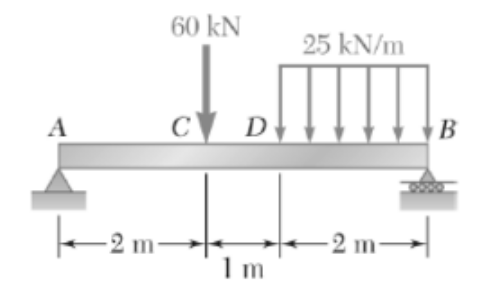For the beam and loading shown, (a) draw the shear and bending-moment diagrams, (b) determine the maximum absolute values of the shear and bending moment.

• ### For the beam and loading shown, (a) draw the shear and bending-moment diagrams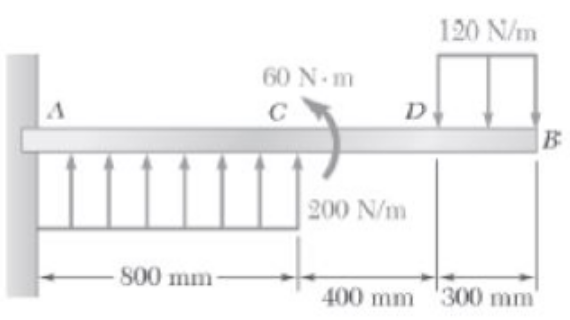For the beam and loading shown, (a) draw the shear and bending-moment diagrams, (b) determine the maximum absolute values of the shear and bending moment.

• ### For the beam and loading shown, (a) draw the shear and bending-moment diagrams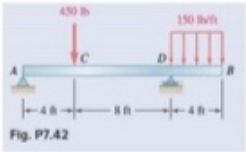For the beam and loading shown, (a) draw the shear and bending-moment diagrams, (b) determine the maximum absolute values of the shear and bending moment.

• ### For the beam and loading shown draw the shear and bending moment diagrams and then find...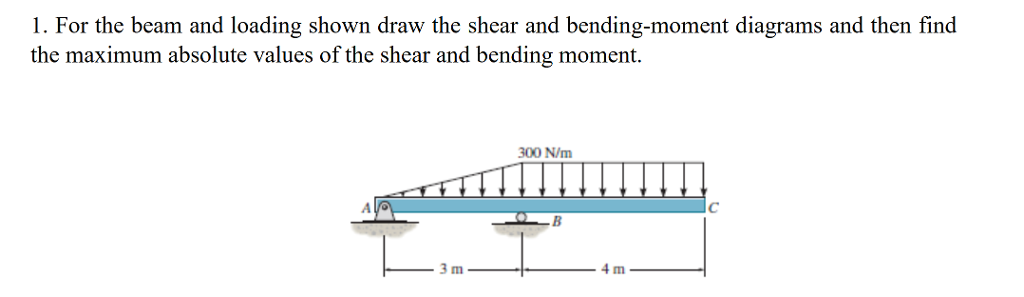For the beam and loading shown draw the shear and bending moment diagrams and then find the maximum absolute values of the shear and bending moment. 1. For the beam and loading shown draw the shear and bending-moment diagrams and then find the maximum absolute values of the shear and bending moment. 300 N/m 4 m

• ### Draw the shear and bending-moment diagrams for the beam and loading shown, and determine the maximum absolute value (a) of the shear, (b) of the bending moment.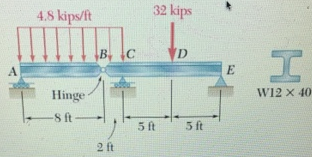Draw the shear and bending-moment diagrams for the beam and loading shown, and determine the maximum absolute value (a) of the shear, (b) of the bending moment.

• ### For the beam and loading shown, (a) draw the shear and bending-moment diagrams, (b) determine the maximum absolute values of the shear and bending moment.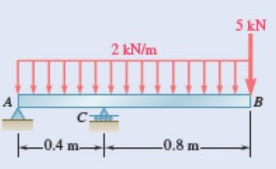7.69 and 7.70 For the beam and loading shown, (a) draw the shear and bending-moment diagrams, (b) determine the maximum absolute values of the shear and bending moment.

• ### 1. For the beam and loading shown, (a) draw the shear and bending-moment diagrams, (b) determine...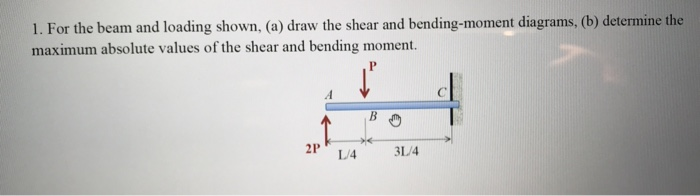1. For the beam and loading shown, (a) draw the shear and bending-moment diagrams, (b) determine the maximum absolute values of the shear and bending moment. L/4 3L4